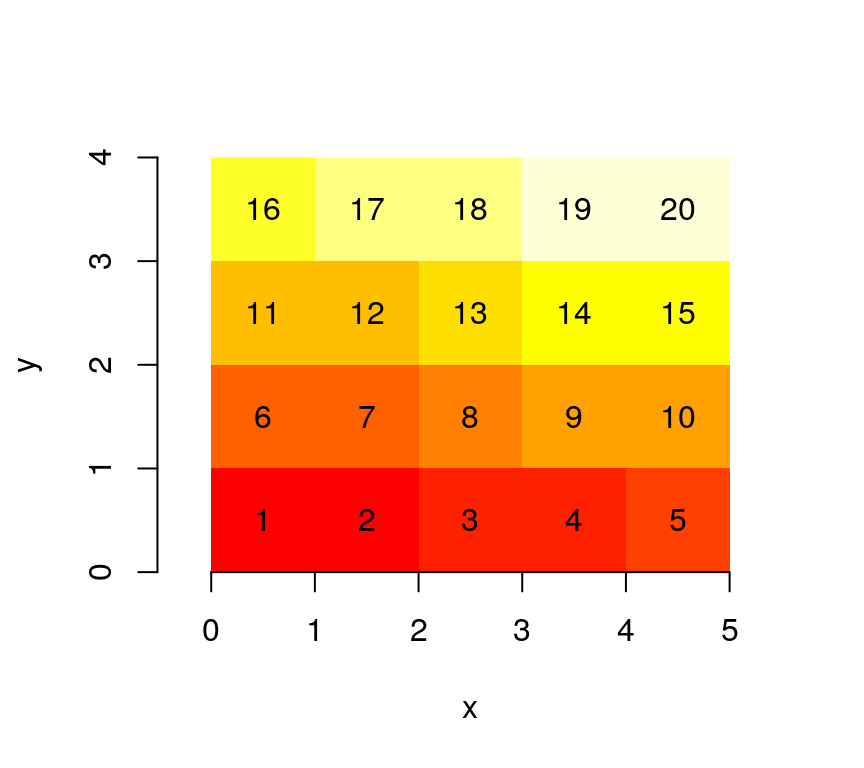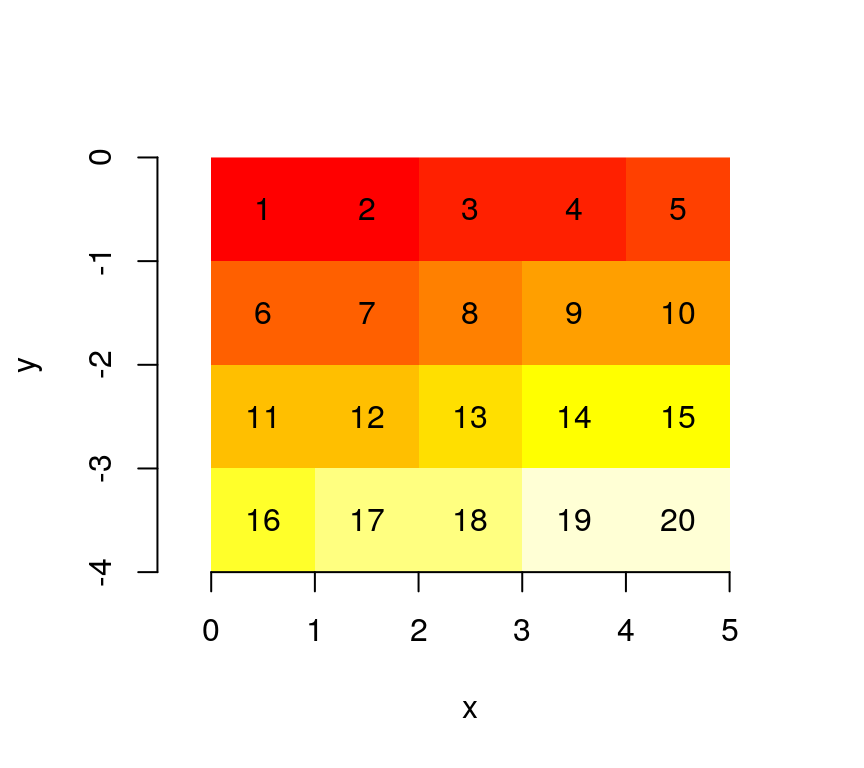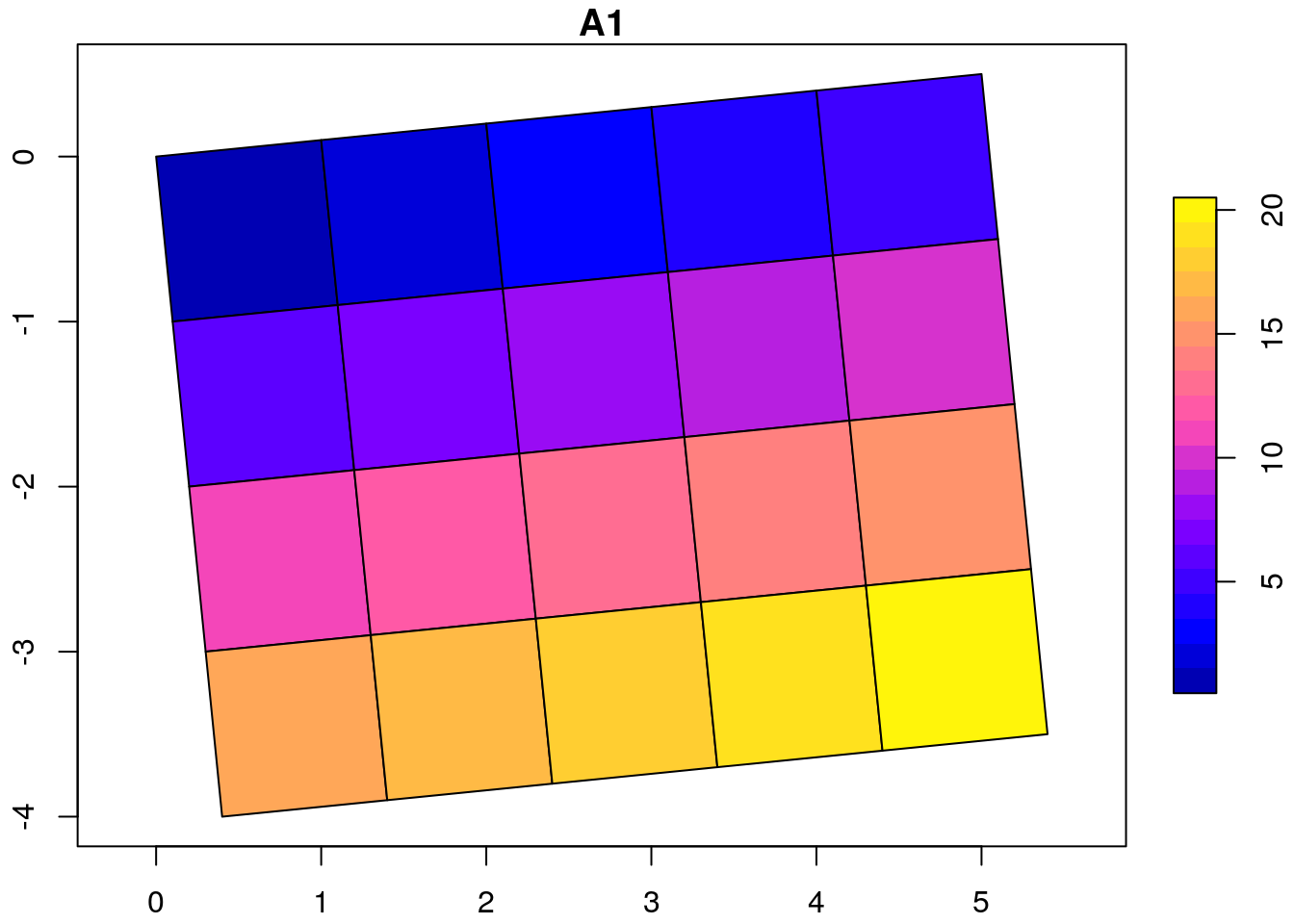This vignette explains the data model of stars objects, illustrated using artificial and real datasets.

## Grid type

### Regular grids

With a very simple file created from a $$4 \times 5$$ matrix

suppressPackageStartupMessages(library(stars))
m = matrix(1:20, nrow = 5, ncol = 4)
dim(m) = c(x = 5, y = 4) # named dim
(s = st_as_stars(m))
## stars object with 2 dimensions and 1 attribute
## attribute(s):
##       A1
##  Min.   : 1.00
##  1st Qu.: 5.75
##  Median :10.50
##  Mean   :10.50
##  3rd Qu.:15.25
##  Max.   :20.00
## dimension(s):
##   from to offset delta refsys point values
## x    1  5      0     1     NA    NA   NULL [x]
## y    1  4      0     1     NA    NA   NULL [y]

we see that

• the rows (5) are mapped to the first dimension, the x-coordinate
• the columns (4) are mapped to the second dimension, the y-coordinate
• the from and to fields of each dimension define a range that corresponds to the array dimension:
dim(s[])
## x y
## 5 4
• offset and delta specify how increasing row and column index maps to x and y coordinate values respectively.

When we plot this object, using the image method for stars objects,

image(s, text_values = TRUE, axes = TRUE)we see that $$(0,0)$$ is the origin of the grid (grid corner), and $$1$$ the coordinate value increase from one index (row, col) to the next. It means that consecutive matrix columns represent grid lines, going from south to north. Grids defined this way are regular: grid cell size is constant everywhere.

Many actual grid datasets have y coordinates (grid rows) going from North to South (top to bottom); this is realised with a negative value for delta. We see that the grid origing $$(0,0)$$ did not change:

attr(s, "dimensions")[]$delta = -1 image(s, text_values = TRUE, axes = TRUE)An example is the GeoTIFF carried in the package, which, as probably all data sources read through GDAL, has a negative delta for the y-coordinate: tif = system.file("tif/L7_ETMs.tif", package = "stars") st_dimensions(read_stars(tif))["y"] ## from to offset delta refsys point values ## y 1 352 9120761 -28.5 +proj=utm +zone=25 +south... FALSE NULL ### Raster attributes, rotated and sheared grids Dimension tables of stars objects carry a raster attribute: str(attr(st_dimensions(s), "raster")) ## List of 3 ##$ affine     : num [1:2] 0 0
##  $dimensions : chr [1:2] "x" "y" ##$ curvilinear: logi FALSE
##  - attr(*, "class")= chr "stars_raster"

which is a list that holds

• dimensions: character, the names of raster dimensions (if any), as opposed to e.g. spectral, temporal or other dimensions
• affine: numeric, the affine parameters
• curvilinear: a logical indicating whether the raster is curvilinear

These fields are needed at this level, because they describe properties of the array at a higher level than individual dimensions do: a pair of dimensions forms a raster, both affine and curvilinear describe how x and y as a pair are derived from grid indexes (see below) when this cannot be done on a per-dimension basis.

With two affine parameters $$a_1$$ and $$a_2$$, $$x$$ and $$y$$ coordinates are derived from (1-based) grid indexes $$i$$ and $$j$$, grid offset values $$o_x$$ and $$o_y$$, and grid cell sizes $$d_x$$ and $$d_y$$ by

$x = o_x + (i-1) d_x + (j-1) a_1$

$y = o_y + (i-1) a_2 + (j-1) d_y$ Clearly, when $$a_1=a_2=0$$, $$x$$ and $$y$$ are entirely derived from their respective index, offset and cellsize.

Note that for integer indexes, the coordinates are that of the starting edge of a grid cell; to get the grid cell center of the top left grid cell (in case of a negative $$d_y$$), use $$i=1.5$$ and $$j=1.5$$.

We can rotate grids by setting $$a_1$$ and $$a_2$$ to a non-zero value:

attr(attr(s, "dimensions"), "raster")$affine = c(0.1, 0.1) plot(st_as_sf(s, as_points = FALSE), axes = TRUE, nbreaks = 20)The rotation angle, in degrees, is atan2(0.1, 1) * 180 / pi ##  5.710593 ### Regular grids: sheared, sheared and rotated Sheared grids are obtained when the two rotation coefficients, $$a_1$$ and $$a_2$$, are unequal: attr(attr(s, "dimensions"), "raster")$affine = c(0.1, 0.2)
plot(st_as_sf(s, as_points = FALSE), axes = TRUE, nbreaks = 20)